#Function Repository Resource:

# KurtosisAround

Calculate the kurtosis of a list along with its uncertainty

Contributed by: Sander Huisman
 ResourceFunction["KurtosisAround"][{x1,x2,x3,…}] gives an Around object describing the kurtosis and corresponding uncertainty of the xi.

## Details

The standard error of the kurtosis is defined as: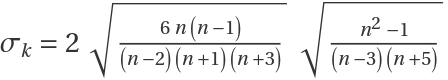,  where n is the number of samples, and the first radical is the standard error of the skewness.
The "Value" property of the Around object is calculated using the built-in Kurtosis function.

## Examples

### Basic Examples (1)

Calculate the kurtosis of some numbers:

 In:=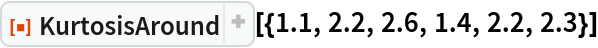Out=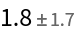### Scope (2)

KurtosisAround works with symbolic expressions:

 In:=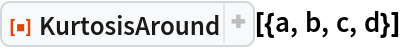Out=KurtosisAround also works with quantities with units, though the answer is dimensionless:

 In:=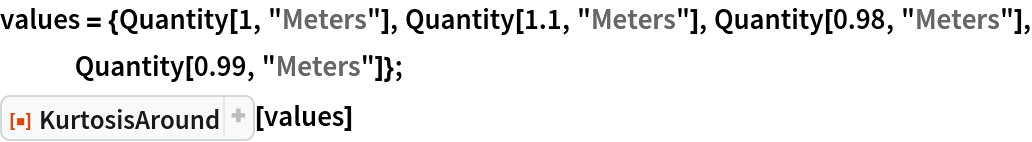Out=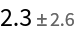The kurtosis is independent of the units:

 In:=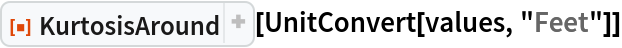Out=### Properties and Relations (1)

The standard error has an asymptote of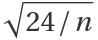where n is the number of data points:

 In:=Out=### Possible Issues (3)

KurtosisAround applied to symbolic expressions can get unwieldy fast:

 In:=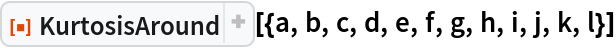Out=At least four value are needed to give a plausible error estimate:

 In:=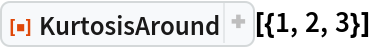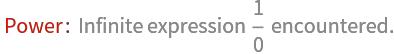Out=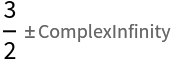Try with 4 values:

 In:=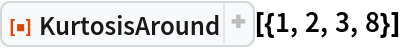Out=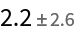Kurtosis can be Indeterminate, in which case there will not be an Around result:

 In:=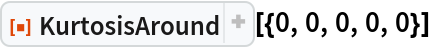Out=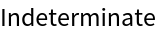### Neat Examples (1)

Study the kurtosis and its uncertainty for a sequence of values and compare it to the theoretical value of the distribution (dashed):

 In:=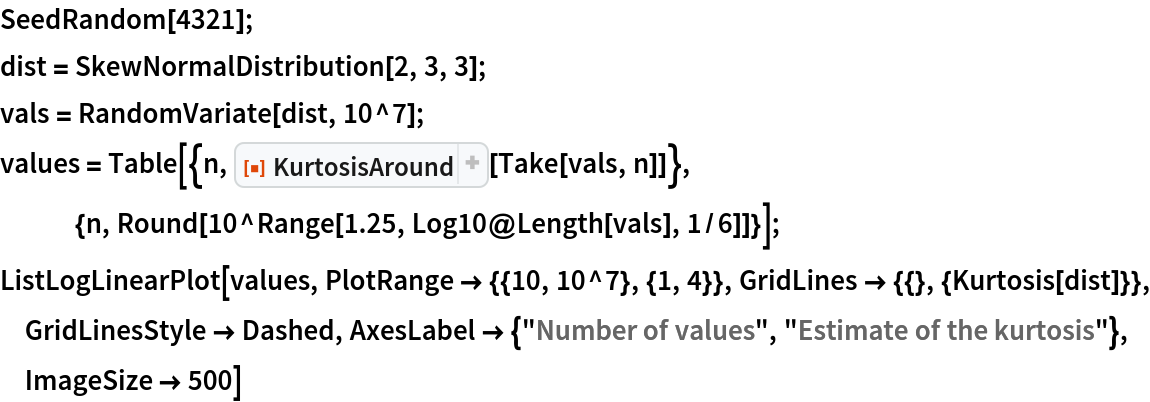Out=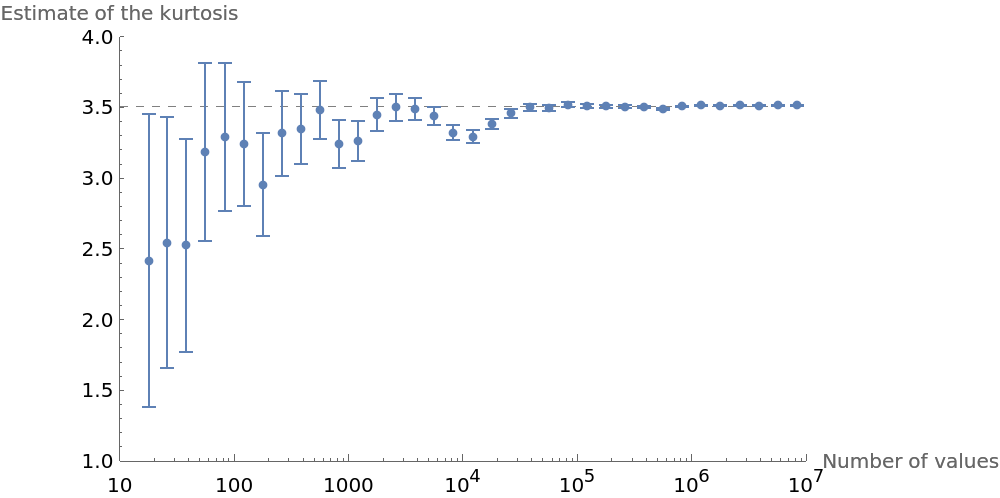SHuisman

## Version History

• 1.0.0 – 21 November 2022